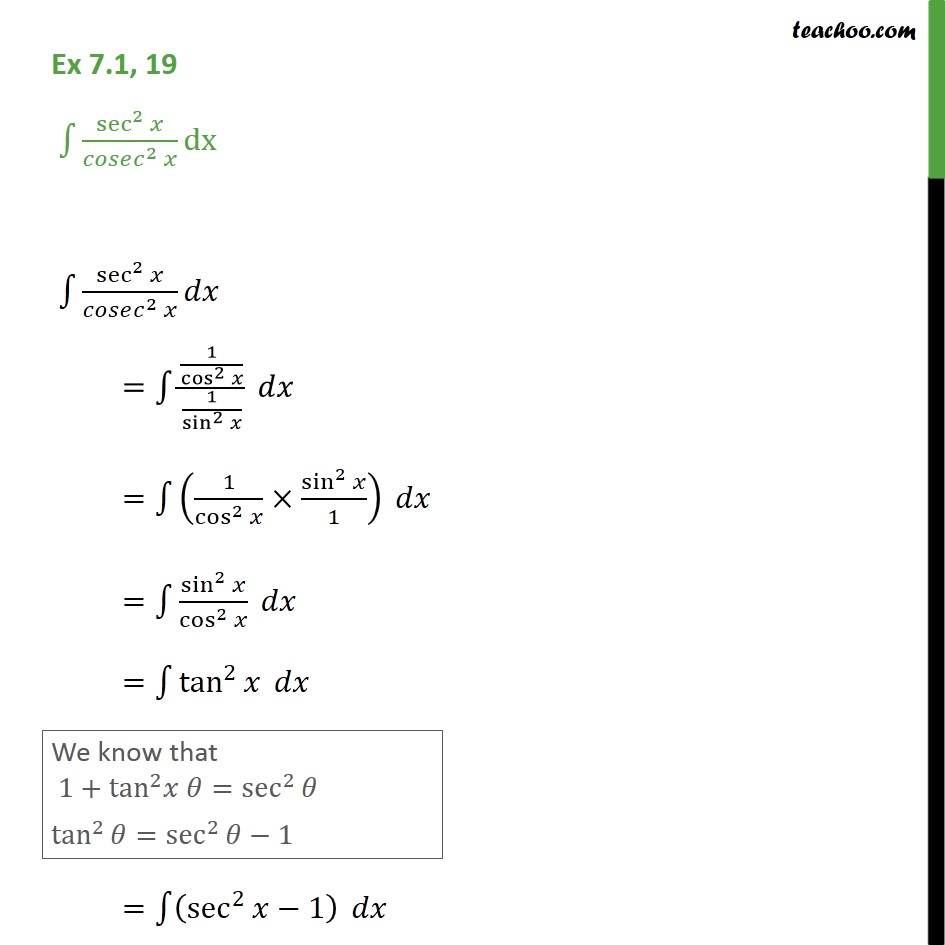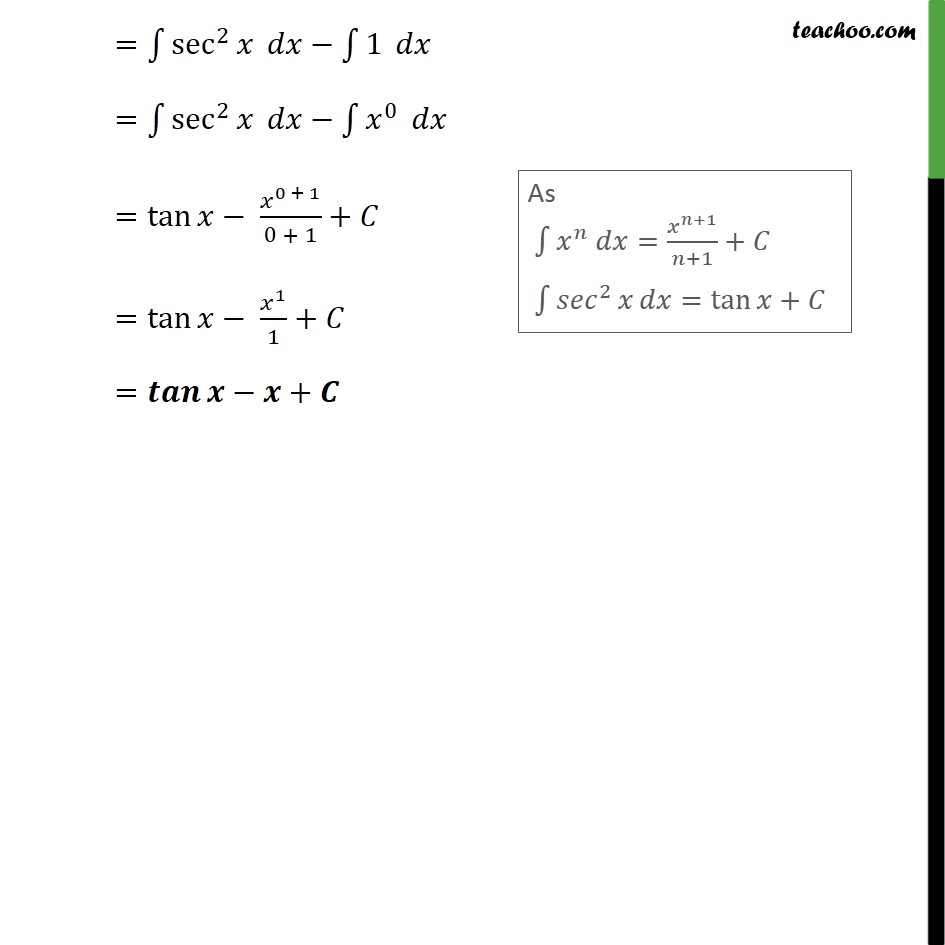Integration Full Chapter Explained - Integration Class 12 - Everything you need1. Chapter 7 Class 12 Integrals
2. Serial order wise
3. Ex 7.1

Transcript

Ex 7.1, 19 sec 2 2 dx sec 2 2 = 1 cos 2 1 sin 2 = 1 cos 2 sin 2 1 = sin 2 cos 2 = tan 2 = sec 2 1 = sec 2 1 = sec 2 0 = tan 0 + 1 0 + 1 + = tan 1 1 + = +

Ex 7.1

Chapter 7 Class 12 Integrals
Serial order wise